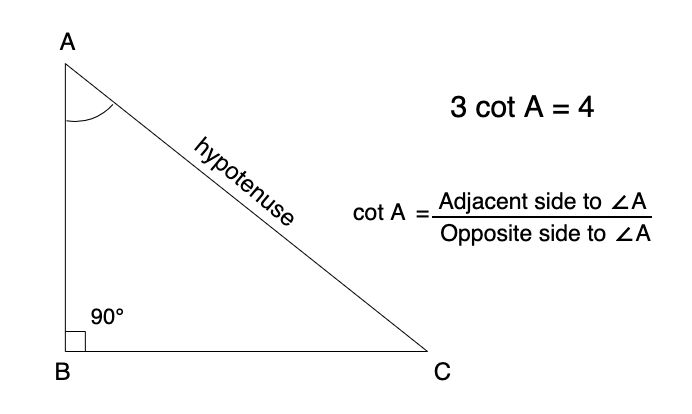# 3 cot A = 4 | Check if (1−tan²A)/(1+tan²A) = cos²A - sin²A | Trigonometry Numerical

Comments

### Question: If 3 cot A = 4, check whether (1−tan²A)/(1+tan²A) = cos²A - sin²A

Consider the following $\triangle ABC$, where $m \angle B = 90 \degree$fig. 1 (drawn from details provided in question)

Important tip: When solving problems of trigonometry, try drawing a right angled triangle with reference to the details given in the question. This can help you visualize and work faster when calculating trigonometric ratios.

Let's start solving this problem by using the given equation $\ 3 \cot A = 4$ and find the values for AB, BC and AC.

\begin{aligned} We \ know & \ that, \\ &3 \cot A = 4 \\ \therefore & \ \cot A = {4 \over 3} \\ \\ From \ \triangle ABC, \\ \ & \cot \ A = {AB \over BC} \quad \bigg[\cot \ A = {\text{Adjacent side to } \angle A \over \text{Opposite side to } \angle A}\bigg] \\ \therefore & \ {AB \over BC} = {4 \over 3} \quad ---(1) \end{aligned}

Hence, from equation (1) we can say $AB = 4x$ and $BC = 3x$

Wondering why we have taken "4x" and "3x" here?

Check this video for understanding it.

As we have already done previously in Trigonometry Numerical | PQ = 12cm and PR = 13cm | Find tan P - cot R and Trigonometry Numerical | 15 cot A = 8 | Find the value of sin A and sec A, let's use the value of AB and BC to find the value of AC by applying Pythagoras Theorem.

\begin{aligned} From \ \triangle &ABC, \ m\angle B = 90\degree \\ \therefore \ AC^2 &= AB^2 + BC^2 \quad [\text{By Pythagoras theorem}] \\ &= (4x)^2 + (3x)^2 \\ &= 16x^2 + 9x^2 \\ &= 25x^2 \\ \therefore \ AC^2 &= (5x)^2 \\ \therefore \ AC &= 5x \end{aligned}

Now, that we have the values of all the sides of $\triangle ABC$, we'll put the values in LHS and RHS and prove that LHS = RHS. Let's start with LHS.### Solving LHS

Firstly finding the value of $\tan A$.

\begin{aligned} From \ &\triangle ABC, \\ \tan \ A &= {\text{Opposite side to m} \angle A \over \text{Adjacent side to m} \angle A} \\ \\ &= {BC \over AB} \\ \\ &= {3x \over 4x} \\ \\ \therefore \ \tan A &= {3 \over 4} \quad --- (2) \\ \end{aligned}

\begin{aligned} LHS &= {1 - \tan^2 A \over 1 + \tan^2A} \\ \\ &= {1 - \Big({3 \over 4}\Big)^2 \over 1 + \Big({3 \over 4}\Big)^2} \quad \big(\text{Putting the values of tan A from equation (2)}\big) \\ \\ &= {1 - {9 \over 16} \over 1 + {9 \over 16}} \\ \\ &= {{16 - 9 \over 16} \over {16 + 9 \over 16}} \\ \\ &= {{16 - 9 \over \cancel{16}} \over {16 + 9 \over \cancel{16}}} \\ \\ &\boxed{\therefore LHS = {7 \over 25}} \quad --- (3) \end{aligned}

### Solving RHS

\begin{aligned} RHS &= \cos^2A - \sin^2A \\ &= \Big({\text{Adjacent side to } \angle A \over Hypotenuse}\Big)^2 - \Big({\text{Opposite side to } \angle A \over Hypotenuse}\Big)^2 \\ \\ &= \Big({AB \over AC}\Big)^2 - \Big({BC \over AC}\Big)^2 \quad (\text{from the figure of } \triangle ABC)\\ \\ &= \Big({4x \over 5x}\Big)^2 - \Big({3x \over 5x}\Big)^2 \\ \\ &= {16x^2 \over 25x^2} - {9x^2 \over 25x^2} \\ \\ &= {7x^2 \over 25x^2} \\ \\ &\boxed{\therefore RHS = {7 \over 25}} \quad ---(4) \end{aligned}

From equation (3) and (4), we can say $LHS = RHS$

$\boxed{\therefore {1 - \tan^2 A \over 1 + \tan^2A} = \cos^2A - \sin^2A}$

Hence, proved.

### Comments:

All comments that you add will await moderation. We'll publish all comments that are topic related, and adhere to our Code of Conduct.

Want to tell us something privately? Contact Us